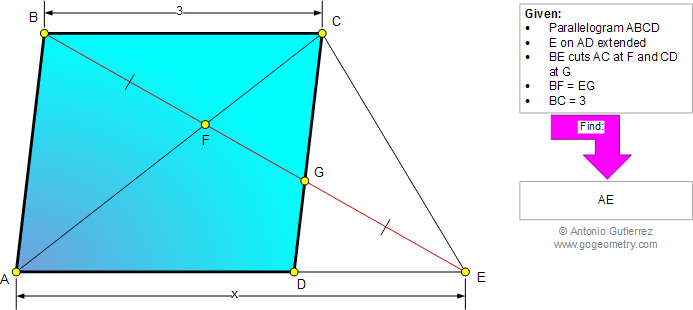###### Online Geometry Problem 864: Parallelogram, Diagonal, Congruence, Similarity, Metric Relations. Level: High School, College, Mathematics Education

 The figure below shows a parallelogram ABCD. E is a point on AD extended. BE cuts AC at F and CD at G. If BF = EG and BC = 3, find AE.Geometry problem solving is one of the most challenging skills for students to learn. When a problem requires auxiliary construction, the difficulty of the problem increases drastically, perhaps because deciding which construction to make is an ill-structured problem. By “construction,” we mean adding geometric figures (points, lines, planes) to a problem figure that wasn’t mentioned as "given."
 Home | Search | Geometry | Problems | All Problems | Open Problems | Visual Index | 10 Problems | 861-870 | Triangles | Parallelogram | Similarity, Ratios, Proportions | Email | Solution / comment | By Antonio Gutierrez